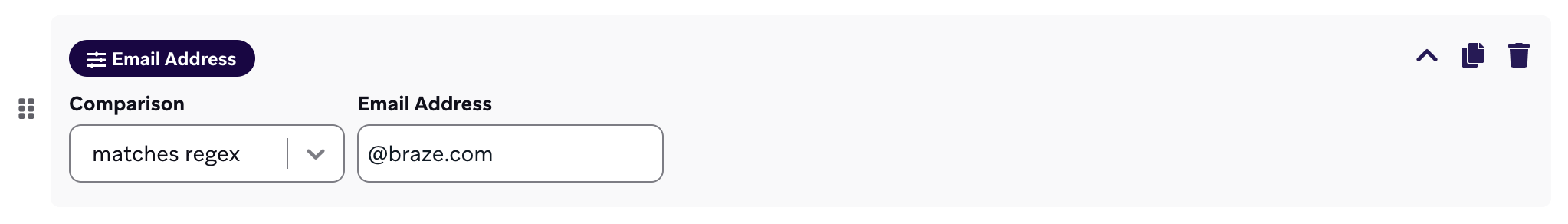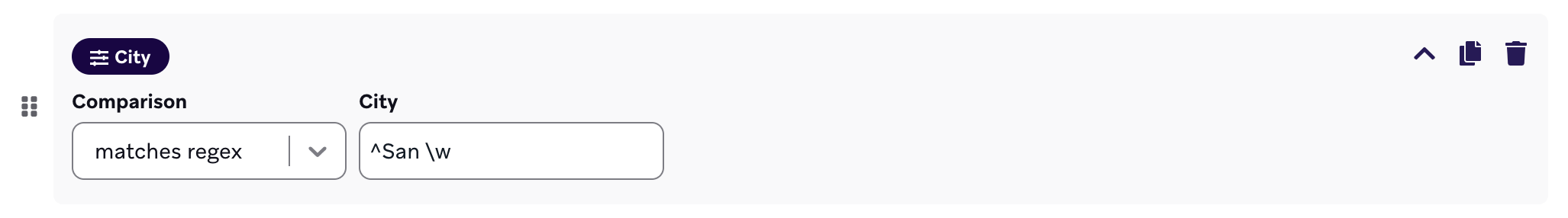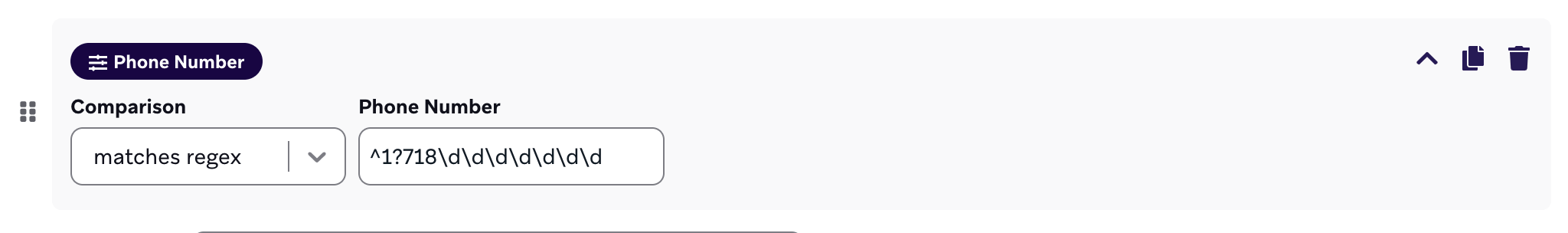# Regular expressions with Braze

Regular expressions, known commonly as regex, is a sequence of characters that define a search pattern. Regular expressions let you do validation of text grouping and find and replace actions.

Regex is used at Braze to give you a more flexible string matching solution in your segmentation and campaign filtering for your target audience.

In the provided video, we show you how regular expressions can be used and tested on the Regex101 site. Below we also offer an inhouse regex tester, a helpful cheatsheet, sample data referenced in the Regex LAB video, as well as some frequently asked questions.

This form allows for basic validation and testing of regular expressions. ​

Regex: ​
/
/gi

Check Value(s):

​ Matched Results:
How do I filter for inbox specific email addresses when segmenting?

Use the email address filter, set it to “matches regex”. Reference the regex for email addresses, [a-zA-Z0-9.+_-]+ @[a-zA-Z0-9.-]+.[a-zA-Z.-]+ where:

• [a-zA-Z0-9.+_-]+

is the beginning of the email address before the ‘@’ character. So the “name” in “name@example.com”.

• [a-zA-Z0-9.-]+

is the first part of the domain. So the “example” in “name@example.com”.

• [a-zA-Z.-]+

is the last part of the domain. So the “com” in “name@example.com”.

How do I filter for email addresses associated to a specific domain?

Say you want to filter for emails ending with @braze.com. You would use the email address filter, set it to matches regex, and enter “@braze.com” in the field.How can I use regex on number strings to filter for values ≥ x or ≤ x?

If you’re searching for values ≥ x, the regex to use would be ^([x-y]|\d{z,})\$ where x-y is the range of numbers (0-9) of the first digit, and z is the one more the number of digits of x.
Example
For values ≥ 50, the regex would then be ^([5-9][0-9]|\d{3,})\$

If you’re searching for values ≤ x, the regex would be ^([x-y]|[a-b])\$ where x-y is the range of numbers (0-9) of the first digit, and a-b is lower bound range of x.
Example
For values ≤ 50, the regex would then be ^([5-9][0-9]|[0-4][0-9])\$

Use the ^ to character to denote what the string starts with and enter the name of you’re trying to specify.

Example
If you’re trying to target users who live in cities that start with “San”, your regex would be ^San \w. In such a case, you would successfully target users from cities like San Francisco, San Diego, San Jose, etc.How to filter for certain phone numbers with regex

Before using regex to filter phone numbers, please take note that numbers logged for user profiles should already be in an E.164 format as specified in our documentation.

Assuming US phone numbers, the regex you’ll want to use is in the format 1?\d\d\d\d\d\d\d\d\d\d, where each “\d” is a digit you want to specify - the first 3 of which would be the area code.

Likewise, the format for UK phone numbers are ^+4\d\d\d\d\d\d\d\d\d\d\d and any other country would be the the respective country code followed by the necessary number of “\d” characters for each remaining digit. So in the case of Lithuania with country code 3, their regex would be ^+3\d\d\d\d\d\d\d\d\d\d.

Example
Let’s say you wanted to filter users by phone number for a specific area code, 718. Use the phone number filter, set it to “matches regex”, and enter ^1?718\d\d\d\d\d\d\d